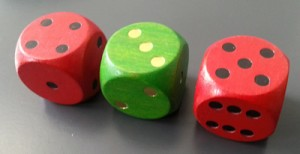Two dice are RED and one is GREEN.Roll the dice and add up the numbers on the two RED dice and then subtract the number on the GREEN.

So if one RED is 4 and the other RED is 5 and the GREEN is 3 we should add together 4 and 5 to make 9 and then subtract the 3 to get a final answer of 6.

Suppose you play a game against a friend. In the Zero-Six game you win if the final score with the 3 dice is zero and she wins if the final score is 6. Is this a fair game? How do you know? What about the Odd-Even game where you win if the final score is odd and she wins if it is even?

You’ll need to roll these dice many times and see what numbers you make each time by doing the addition and subtraction.

In this game it would be good to find out:-

• what are the final answers by doing the addition and subtraction each time?
• what are all the different possible numbers?
• is there a good way of making sure you find them all?
• how will you record what you’ve found out?

Now have a go!

Look at your results and write down some questions that you could ask about them. For example, do any of them have the same answers? If so, why?

Then you could ask yourself, “I wonder what would happen if, instead, I …?”

Make up your own games with different rules and decide whether your game is fair or not.

1.Cup Xalabile says: